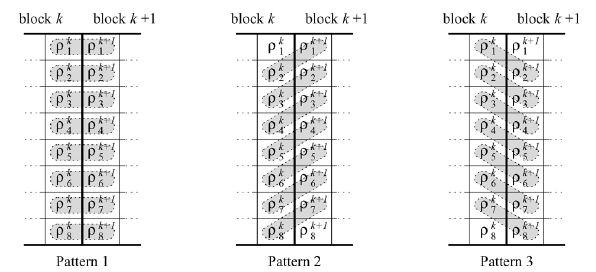# 2006-IEEE-Recovering DC coefficients in block-based DCT

【注】此论文中谈论的图像均为像素值在 $(0,255)$ 范围内的 RGB 图像，定义点 $(i,j)$像素值$f(i,j)$

## 1. 拉普拉斯分布特性

$\begin{array}{c} \{(i-1,j-1),(i-1,j),(i-1,j+1),(i,j-1),(i,j+1),(i+1,j-1),(i+1,j),(i+1,j+1)\} \end{array}$

$\begin{array}{c} Q(i,j)=\{f(i-1,j-1),f(i-1,j),f(i-1,j+1),f(i,j-1),f(i,j+1),f(i+1,j-1),f(i+1,j),f(i+1,j+1)\} \end{array}$

$\begin{array}{c} f(i,j) - q(i,j) & \forall i, j, q(i,j) \in Q(i,j) \end{array}$

## 2. JPEG 中的 DCT 和 IDCT 公式

JPEG 中采用的是 DCT-II 公式。其中

$\begin{array}{c} C(x) = \left\{ \begin{array}{c} \sqrt{\frac{1}{8}} & x = 0 \\ \frac{1}{2} & x \neq 0 \end{array} \right. \end{array}$

### 2.1 DCT 公式

$\begin{array}{c} F(u,v) = C(u) C(v) \sum_{i=0}^7 \sum_{j=0}^7 f(i,j) \cdot \cos{\frac{2i+1}{16} u \pi} \cos{\frac{2j+1}{16} v \pi} \end{array}$

### 2.2 IDCT 公式

$\begin{array}{c} \hat{f}(i,j) = \sum_{u=0}^7 \sum_{v=0}^7 C(u) C(v) F(u,v) \cdot \cos{\frac{2i+1}{16} u \pi} \cos{\frac{2j+1}{16} v \pi} \end{array}$

## 3. AC 系数预测 DC 系数

### 3.1 原理

$\begin{array}{c} p^{(k)} = \rho^{(k)} + \frac{1}{N^2} c_{1,1}^{(k)} \\ p^{(k+1)} = \rho^{(k+1)} + \frac{1}{N^2} c_{1,1}^{(k+1)} \end{array}$

$\begin{array}{c} & p^{(k+1)} & \approx & p^{(k)} \\ \Rightarrow & \rho^{(k+1)} + \frac{1}{N^2} c_{1,1}^{(k+1)} & \approx & \rho^{(k)} + \frac{1}{N^2} c_{1,1}^{(k)} \\ \Rightarrow & c_{1,1}^{(k+1)} & \approx & c_{1,1}^{(k)} + N^2 (\rho^{(k)} - \rho^{(k+1)}) \end{array}$

### 3.2 方式$\begin{array}{c} c_{1,1}^{(k+1)} \approx c_{1,1}^{(k)} + \frac{N^2}{8} \sum_{i=1}^8 (\rho^{(k)}_i - \rho^{(k+1)}_i) \end{array}$

## 4. JPEG 压缩中 DC 系数与 AC 系数之间的制约关系

• 若 AC 系数的可变化范围大，则说明 DC 系数较小。
• 若 DC 系数较大，则说明 AC 系数的可变化范围小。

DC 系数和 AC 系数的取值范围不同，且最值不能同时取到。比如：

• DC 系数的取值范围为 $[-1024, 1024]$
• 某些 AC 系数的取值范围为 $[-420.3863, 420.3863]$